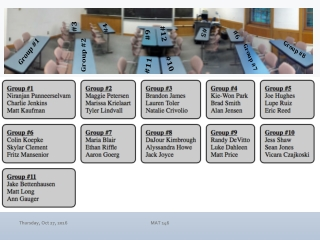DownloadDownload PresentationSeating by Group

# Seating by Group

Télécharger la présentation## Seating by Group

- - - - - - - - - - - - - - - - - - - - - - - - - - - E N D - - - - - - - - - - - - - - - - - - - - - - - - - - -
##### Presentation Transcript

1. Seating by Group MAT 146

2. Calculus II (MAT 146)Dr. Day Thursday, Oct 27, 2016 • More Integration Applications • Arc Length of a Curve (8.1) • Probability (8.5) • Differential Equations (Ch 9) • What is a differential equation? • Solving Differential Equations • Visual Solutions • Numerical Solutions • Analytical Solutions • Applications of Differential Equations • Infinite Series (Ch 11) • Integration Applications • Area Between Curves (6.1) • Average Value of a Function (6.5) • Volumes of Solids (6.2, 6.3) • Created by Rotations • Created using Cross Sections • Methods of Integration • U-substitution (5.5) • Integration by Parts (7.1) • Trig Integrals (7.2) • Trig Substitution (7.3) • Partial-Fraction Decomposition (7.4) • Putting it All Together: Strategies! (7.5) • Improper Integrals (7.8) MAT 146 Test #2: Thursday & Friday, Williams Hall Room 128 Review Session: Wednesday, 6:15-7:30, STV 352

3. MAT 146

4. MAT 146

5. Solving Differential Equations Solve for y: y’ = −y2 MAT 146

6. Separable Differential Equations Solve for y: y’ = 3xy MAT 146

7. Separable Differential Equations Solve for z: dz/dx+ 5ex+z = 0 MAT 146

8. Separable Differential Equations Solve for P using separation of variables, knowing (1,2) satisfies your solution: dP/dt = (2Pt)1/2 MAT 146

9. Separable Differential Equations Solve for y if: dy/dx= x−xyand y(0)=3 MAT 146

10. Applications! Rate of change of a population P, with respect to time t, is proportional to the population itself. MAT 146

11. Rate of change of the population is proportional to the population itself. Slope Fields Euler’s Method Separable DEs MAT 146

12. Population Growth Suppose a population increases by 3% each year and that there are P=100 organisms initially present (at t=0). Write a differential equation to describe this population growth and then solve for P. MAT 146

13. Separable DEs MAT 146

14. Applications! The radioactive isotope Carbon-14 exhibits exponential decay. That is, the rate of change of the amount present (A) with respect to time (t) is proportional to the amount present (A). MAT 146

15. Exponential Decay The radioactive isotope Carbon-14 exhibits exponential decay. That is, the rate of change of the amount present (C) with respect to time (t) is proportional to the amount present (C). Carbon-14 has a half-life of 5730 years Write and solve a differential equation to determine the function C(t) to represent the amount, C, of carbon-14 present, with respect to time (t in years), if we know that 20 grams were present initially. Use C(t) to determine the amount present after 250 years. MAT 146

16. Applications! MAT 146

17. Applications! The security staff at a rock concert found a dead body in a mezzanine restroom, the apparent victim of a fatal shooting. They alert the police who arrive at precisely 12 midnight. At that instant, the body’s temperature is 91º F; by 1:30 a.m., 90 minutes later, the body’s temperature has dropped to 82º F. Noting that the thermostat in the restroom was set to maintain a constant temperature of 69º F, and assuming the the victim’s temperature was 98.6º F when she was shot, determine the time, to the nearest minute, that the fatal shooting occurred. Assume that the victim died instantly and that Newton’s Law of Cooling holds. Show all appropriate evidence to support your solution. MAT 146

18. Applications! MAT 146

19. MAT 146

20. MAT 146

21. Applications! Mixtures A tank contains 2000 L of brine with 30 kg of dissolved salt. A solution enters the tank at a rate of 20 L/min with 0.25 kg of salt per L . The solution is kept thoroughly mixed and drains from the tank at the same rate. How much salt is in the tank after t minutes? After 60 minutes? MAT 146

22. Applications! Mixtures A tank contains 1000 L of brine with 15 kg of dissolved salt. Pure water enters the tank at a rate of 10 L/min. The solution is kept thoroughly mixed and drains from the tank at the same rate. How much salt is in the tank after t minutes? After 20 minutes? MAT 146

23. Application: Ice Growth Details . . . details . . . details! https://plus.maths.org/content/teacher-package-differential-equations MAT 146

24. Applications! Spreading a Rumor: Suppose that y represents the number of people that know a rumor at time t and that there are M people in the population. For these parameters, one model for the spread of the rumor is that “the rate at which the rumor is spread is proportional to the product of those who have heard the rumor and those who have not heard it.” MAT 146

25. Xeno’s Paradox • I’m 20 feet from the door. I walk half the distance to the door. I stop and record the distance I walked. I now repeat this action from my new starting point. • Distances Walked: • Running Sums of Distances Walked: • Total Distance Walked: MAT 146